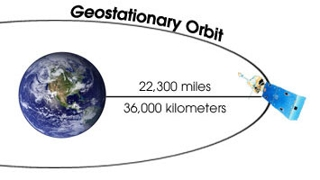Physics

# Explain on Geo-stationary Satellites

A geo-stationary satellite is a particular type used in television and telephone communications. A number of communication satellites which appear to remain in fixed positions at a specified height above the equator are called synchronous satellites or geo-stationary satellites. Some television programmes or events occuring in other countries are often transmitted ‘live’ with the help of these satellites.

For a satellite to appear fixed at a position above a certain place on the Earth, its orbital period around the Earth must be exactly equal to the rotational period of the Earth about its axis.

Consider a satellite of mass m moving in a circular orbit around the Earth at a distance rfrom the centre of the Earth. For synchronisation, its period of revolution around the Earth must be equal to the period of rotation of the Earth (ie) 1 day = 24 hr = 86400 seconds.

The speed of the satellite in its orbit is:

v = Circumference of orbit/Time period

v = 2πr/T

The centripetal force F = mv2/r

So, F = (4mπ2r)/T2

The gravitational force on the satellite due to the earth is: F = GMm/r2Substituting T = 86400 s, R = 6400 km and g = 9.8 m/s2. the radius of the orbit of geo-stationary satellite is calculated as 42400 km.

The height of the geo-stationary satellite above the surface of the Earth is h = r – R = 36000 km.

If a satellite is parked at this height, it appears to be stationary. Three satellites spaced at 120° intervals each above Atlantic. Pacific and Indian oceans provide a worldwide communication network